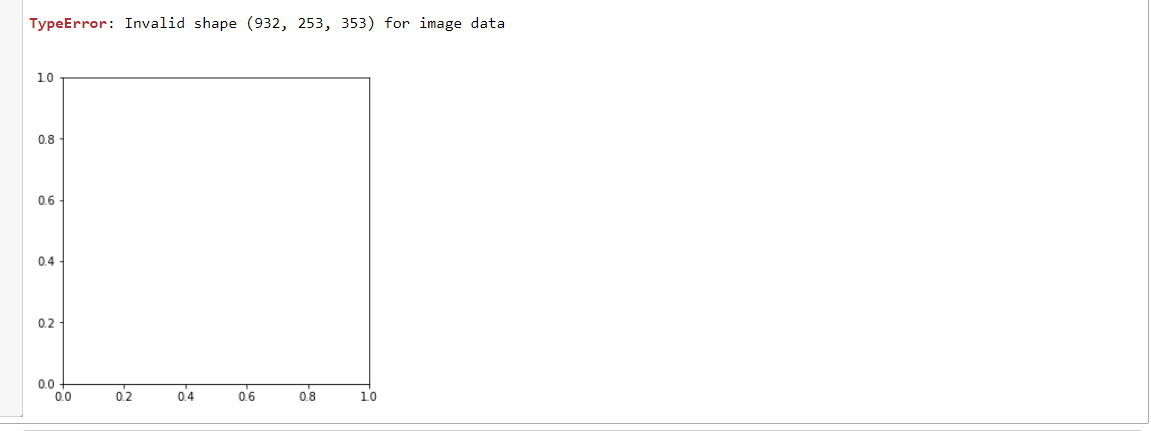Note: Functions listed with a ✔ are custom functions

#### DICOM Datasets

Here is a list of 3 DICOM datasets that you can play around with. Each of these 3 datasets have different attributes and shows how there can be a vast difference in what is contained in different DICOM datasets.

• the SIIM_SMALL dataset ((250 DICOM files, ~30MB) is conveniently provided in the fastai library but is limited in some of its attributes for example it does not have RescaleIntercept or RescaleSlope and its pixel range is limited in the range of 0 and 255
• Kaggle has an easily accessible (437MB) CT medical image dataset from the cancer imaging archive. The dataset consists of 100 images (512px by 512px) with pixel ranges from -2000 to +2000
#Load the dependancies
from fastai.basics import *
from fastai.callback.all import *
from fastai.vision.all import *
from fastai.medical.imaging import *

import pydicom
import seaborn as sns
matplotlib.rcParams['image.cmap'] = 'bone'
from matplotlib.colors import ListedColormap, LinearSegmentedColormap


The SIIM_SMALL dataset is a DICOM dataset where each DICOM file has a pixel_array that contains 1 image. In this case the show function within fastai.medical.imaging conveniently displays the image

source = untar_data(URLs.SIIM_SMALL)
items = get_dicom_files(source)
patient1.show()

1 frame per fileLoading an image from the CT medical image dataset which also contains 1 frame per DICOM file. This image is a slice of a CT scan looking at the lungs with the heart in the middle.

csource = Path('C:/PillView/NIH/data/dicoms')
citems = get_dicom_files(csource)
patient2.show()

1 frame per fileHowever what if a DICOM dataset has multiple frames per DICOM file

The Thyroid Segmentation in Ultrasonography Dataset is a dataset where each DICOMfile has multiple frames per file. Using the same format as above to view an image:

tsource = Path('D:/Datasets/thyroid')
titems = get_dicom_files(tsource)
#patient3.show()


This will result in a TypeError because the current show function does not have a means of displaying files with multiple framesCustomizing the show function now checks to see if the file contains more than 1 frame and then displays the image accordingly. You can also choose how many frames to view (the default is 1). It was also noted that the show_images function does not accept colormaps and hence that function also had to be slightly modified

#updating to handle colormaps
@delegates(subplots)
def show_images(ims, nrows=1, ncols=None, titles=None, cmap=None, **kwargs):
"Show all images ims as subplots with rows using titles"
if ncols is None: ncols = int(math.ceil(len(ims)/nrows))
if titles is None: titles = [None]*len(ims)
axs = subplots(nrows, ncols, **kwargs).flat
for im,t,ax in zip(ims, titles, axs): show_image(im, ax=ax, title=t, cmap=cmap)

#updating to handle multiple frames
@patch
@delegates(show_image, show_images)
def show(self:DcmDataset, frames=1, scale=True, cmap=plt.cm.bone, min_px=-1100, max_px=None, **kwargs):
px = (self.windowed(*scale) if isinstance(scale,tuple)
else self.hist_scaled(min_px=min_px,max_px=max_px,brks=scale) if isinstance(scale,(ndarray,Tensor))
else self.hist_scaled(min_px=min_px,max_px=max_px) if scale
else self.scaled_px)
if px.ndim > 2:
gh=[]
p = px.shape; print(f'{p} frames per file')
for i in range(frames): u = px[i]; gh.append(u)
show_images(gh, cmap=cmap, **kwargs)
else:
print('1 frame per file')
show_image(px, cmap=cmap, **kwargs)

patient3.show(10)

932 frames per fileThe images now display the number of frames specified as well as how many frames there are in each file. It also now allows a cmap to be passed in.

patient3.show(10, cmap=plt.cm.ocean)

932 frames per fileThis function also works when each DICOM file only has 1 frame

patient2.show()

1 frame per file##### Saving files from multiple frames

The Thyroid segmentation dataset is broken down into 2 folders each containing 16 .dcm files each. It would be good to know what the total number of frames are within the dataset.

For this we use a custom function to get the total number of frames in the dataset and how many frames there are in each file

def get_num_frames(source):
"""Get the number of frames in each DICOM"""
"""Some DICOMs have multiple frames and this function helps to find the total number of frames in a DICOM dataset """
frame_list = []
h = get_dicom_files(source)
for i, path in enumerate(h):
test_im = h[i]
try:
v = int(j.NumberOfFrames)
except:
v=1
frame_list.append(v)
sl = sum(frame_list); ll = L(frame_list)
return sl, ll

get_num_frames(tsource)

(31304, (#33) [932,942,1058,1120,958,1064,1134,1060,928,892...])

In this case there are a total of 31304 frames within the dataset with each file having between 800 to 1100 frames. To view a range of frames:

gh = []
for i in range(0,100):
u = patient3.pixel_array[i,:,:]
gh.append(u)
show_images(gh, nrows=10, ncols=10)